# How to generalize (algorithmically)

A couple of months ago I taught an introduction to statistical learning theory. I took inspiration from two very good introductory books on SLT: “Foundations of ML”, and “Understanding Machine Learning: From Theory to Algorithms”. I also covered some classical results about nearest neighbors from the seminal book “A Probabilistic Theory of Pattern Recognition”. The lectures were recorded and I put the videos on the youtube channel. Sadly I still did not learn the basic lesson to stick to a black pen when teaching on a whiteboard, so at (many) times it is hard to read what I write. I also got sick after the first lecture so my voice is quite weak in the following lectures, making it hard to hear too… Despite these fatal flaws some people still found the material useful, so check it out if you are interested. Below I give a rough syllabus for the lectures, and I also give some notes on “algorithmic generalization” which was in my opinion the highlight of the lectures (you can also check my earlier notes on SLT here and here).

Syllabus

Lecture 1: Introduction to the statistical learning theory framework, its basic question (sample complexity) and its canonical settings (linear classification, linear regression, logistic regression, SVM, neural networks). Two basic methods for learning: (i) Empirical Risk Minimization, (ii) Nearest neighbor classification.

Lecture 2: Uniform law of large numbers approach to control the sample complexity of ERM (includes a brief reminder of concentration inequalities). Application: analysis of bounded regression (includes the non-standard topic of type/cotype and how it relates to different regularizations such as in LASSO).

Lecture 3: Reminder of the first two lectures and relation with the famous VC dimension. How to generalize beyond uniform law of large numbers: stability and robustness approaches (see below).

Lecture 4: How to generalize beyond uniform law of large numbers: information theoretic perspective (see below), PAC-Bayes, and online learning. Brief discussion of margin theory, and an introduction to modern questions in robust machine learning.

Some notes on algorithmic generalization

Let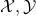be input/output spaces. Let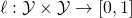be a loss function,a probability measure supported on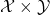, anda learning rule (in wordstakes as input a dataset of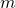examples, and output a mapping from-inputs to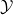-outputs). With a slight abuse of notation, forand, we write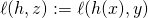. We define the generalization ofonby:In words, if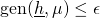then we expect the empirical performance of the learned classifierto be representative of its performance on a fresh out-of-sample data point, up to an additive. The whole difficulty of course is that the empirical evaluation is done with the same dataset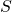that is used for training, leading to non-trivial dependencies. We should also note that in many situations one might be interested in the two-sided version of the generalization, as well as high probability bounds instead of bounds in expectation. For simplicity we focus on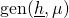here.

The most classical approach to controlling generalization, which we covered in details in previous notes, is via uniform law of large numbers. More precisely assuming that the range of the learning rule is some hypothesis class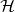one trivially has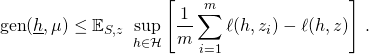However this approach might be too coarse when the learning rule is searching through a potentially huge space of hypothesis (such as in the case of neural networks). Certainly such uniform bound has no chance of explaining why neural networks with billions of parameters would generalize with a data set of merely millions of examples. For this one has to use algorithm-based arguments.

Stability

The classical example of algorithmic generalization is due to Bousquet and Elisseeff 2002. It is a simple rewriting of the generalization as a stability notion: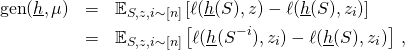where. This viewpoint can be quite enlightening. For example in the uniform law of large numbers view, regularization enforces small capacity, while in the stability view we see that regularization ensures that the output hypothesis is not too brittle (this was covered in some details in the previous notes).

Robustness

The next approach I would like to discuss is related to deep questions about current machine learning methods. One of the outstanding problem in machine learning is that current algorithms are not robust to even mild shift of distribution at test time. Intuitively this lack of robustness seem to indicate a lack of generalization. Can we formalize this intuition? I will now give one such formal link between robustness and generalization due to Xu and Mannor 2010, which shows the reverse direction (robustness implies generalization). At some level robustness can be viewed as a “stability at test time” (while in Bousquet and Elisseeff we care about “stability at training time”).

Xu and Mannor define-robustness as follows: assume thatcan be partitioned intosets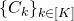such that ifandare in the same setthenA good example to have in mind would be a binary classifier with large margin, in which casecorresponds to the covering number ofat the scale given by the margin. Another (related) example would be regression with a Lipschitz function. In both caseswould be typically exponential in the dimension of. The key result of Xu and Mannor that we prove next is a generalization bound of order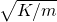. In any situation of interest this seems to me to be a pretty weak bound, yet on the other hand I find the framework to be very pretty and it is of topical interest. I would be surprised if this was the end of the road in the space of “generalization and robustness”.

Theorem (Xu and Mannor 2010):

A-robust learning rule satisfiesProof: Letand note that. Now one has for a robust:It only remains to observe that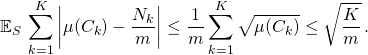Information theoretic perspective

Why do we think that a lack of robustness indicate a lack of generalization? Well it seems to me that a basic issue could simply be that the dataset was memorized by the neural network (which be a very non-robust way to learn). If true then one could basically find all the information about the data in the weights of the neural network. Again, can we prove at least the opposite direction, that is if the output hypothesis does not retain much information from the dataset then it must generalize. This is exactly what Russo and Zou 2016, where they use the mutual informationas a measure of the “information” retained by the trained hypothesis about the dataset. More precisely they show the following result:

Theorem (Russo and Zou 2016):Note that here we have assumed that the codomain of the learning ruleconsists of deterministic maps from inputs to outputs, in which case the mutual informationis simply the entropy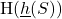. However the proof below also applies to the case where the codomain of the learning rule consists of probability measures, see e.g., Xu and Raginsky 2017. Let us now conclude this (long) post with the proof of the above theorem.

The key point is very simple: one can view generalization as a decoupling property by writing:where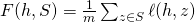.

Now the theorem follows straightforwardly (if one knows Hoeffding’s lemma) from an application of the following beautiful lemma:

Lemma:

Let. Let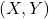be random variables inand,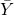be mutually independent copies of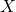and. Assume that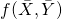is-subgaussian (i.e.,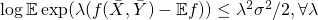) thenProof: The mutual information is equal to the relative entropy between the distribution ofand the distribution of. Recall also the variational representation of the relative entropy which is that the mapis the convex conjugate of the log-partition function. In particular one has a lower bound on the mutual information for any such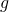which means:Now it only remains to use the definition of subgaussianity, that is take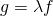, and optimize over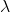.

This entry was posted in Machine learning. Bookmark the permalink.

## 20 Responses to "How to generalize (algorithmically)"

••••••••••••••••••••